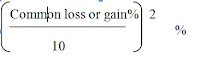# Loss - Shortcut Methods

1. Gain = Selling Price(S.P.) - Cost Price(C.P)
2. Loss = C.P. - S.P.
3. Gain % = Gain * 100 / C.P.
4. Loss % = Loss * 100 / C.P.
5. S.P. = (100+Gain%)/100*C.P.
6. S.P. = (100-Loss%)/100*C.P.
Short cut Methods:

1. By selling an article for Rs. X, a man loses l%. At what price should he sell it to gain y%?       (or)
A man lost l% by selling an article for Rs. X. What percent shall he gain or lose by selling it for Rs. Y?
(100 – loss%) : 1st S.P. = (100 + gain%) : 2nd S.P.
2. A man sold two articles for Rs. X each. On one he gains y% while on the other he loses y%. How much does he gain or lose in the whole transaction?
In such a question, there is always a lose. The selling price is immaterial.
Formula for loss %=3. A discount dealer professes to sell his goods at cost price but uses a weight of 960 gms. For a kg weight. Find his gain percent.

Formula: Gain % =